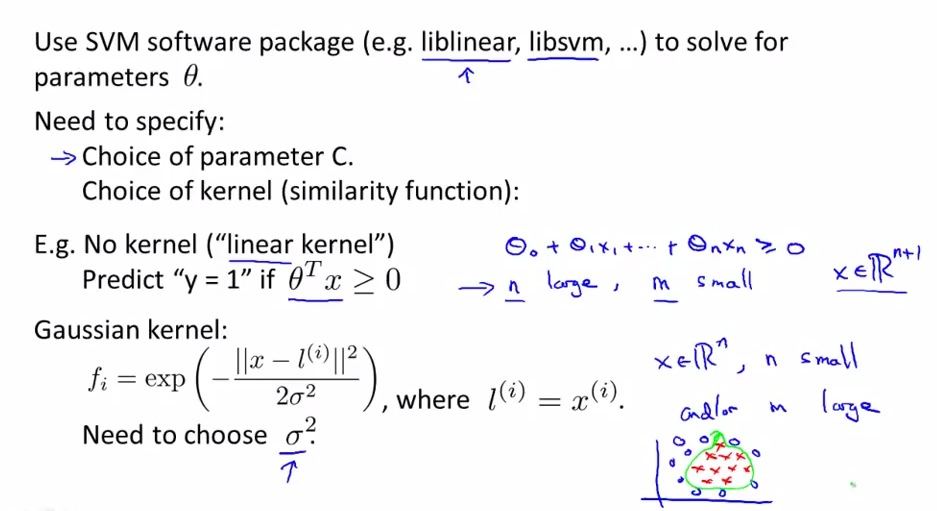• Not recommend to write own code of learning algorithm. almost none of scientist nowaday code its own inverse matrices, multiply, and code its own SVM
• use library that optimized for SVM, such as liblinear and libsvm, two of the most andrew used.
• Sometimes people doesn't want to use kernel. So they are using "SVM with no kernel", that is linear kernel.
• libllinear can do linear kernel, with logics behind this is where we have n huge and m small, we don't want to risk overfitting. And just have svm that regulaize parameters.
• libsvm on the other hand, using Gaussian kernel where's n small m large.
• Simple decision boundary will be enough if we have lot of parameters, but small amount of data. This case will help us avoid overcomplex parameters without enough data
• We maybe didn't need to code ourself but it is necessary for us to modify C value and sigma value.
• There will be a tutorial to walkthrough to choose between linear kernel or gaussian kernel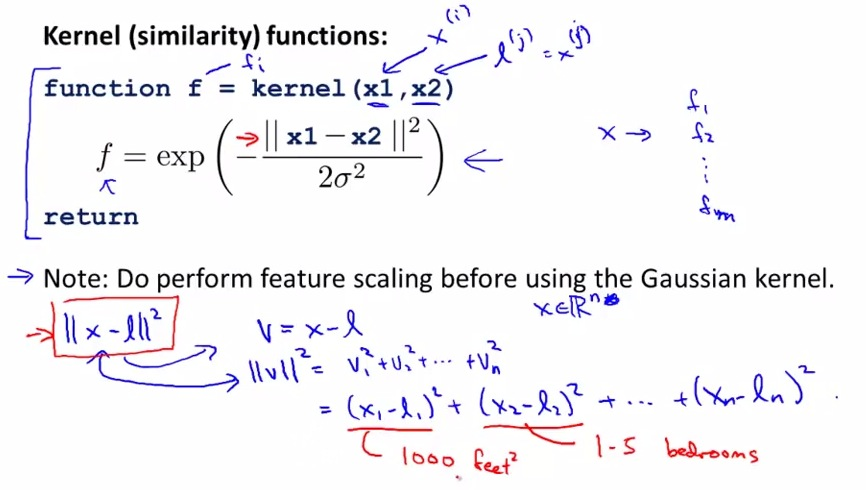• Now libsvm sometimes not provided f function, so we should write it ourself, f the Gaussian kernel.
• But often many svm library already included Gaussian, as linear kernel and Gaussian kernel, as the the two are both most popular kernel in SVM.
• the libsvm will eventually iterate from f1 to fm to calculate similarity to xi
• perform feature scaling so one particular feature do not overly weight (nullify the other features) in example of size of houses nullify bedroom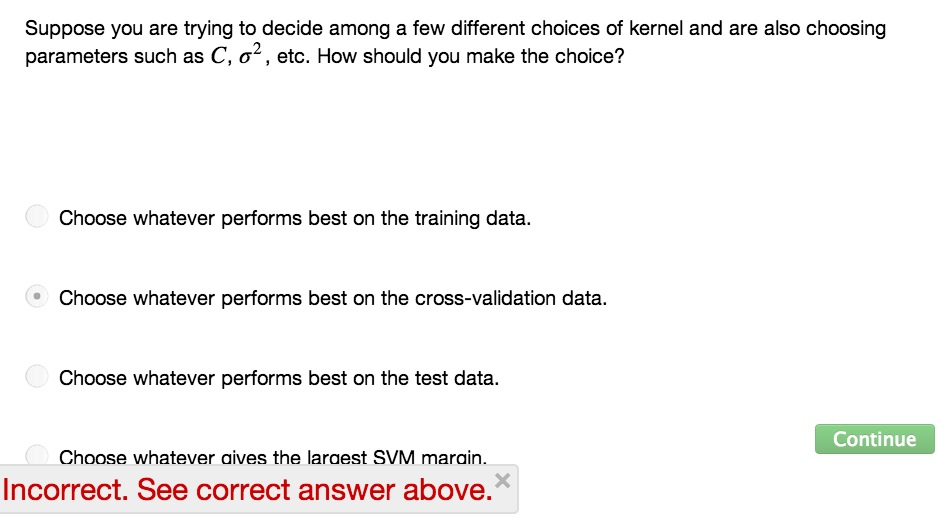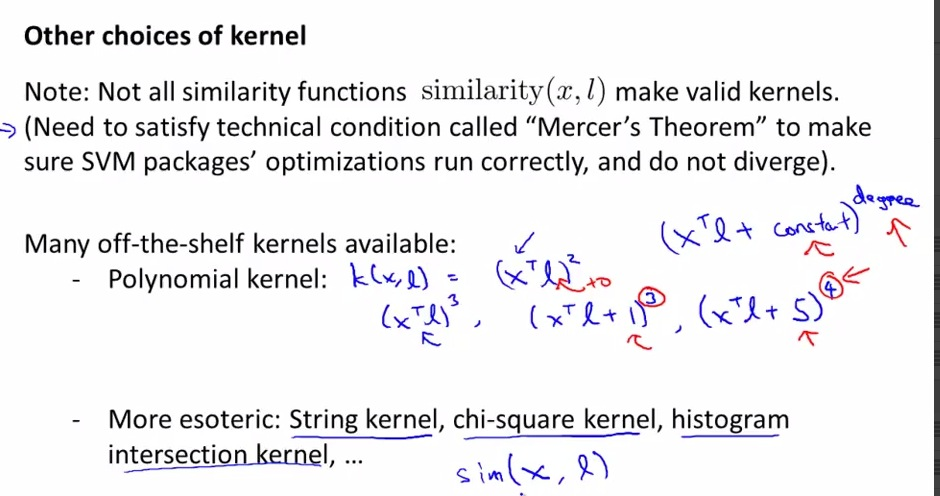• use mercer's theorem to avoid invalid kernels. SVM back then by numerical computing, wants to be computed efficiently. So by generalizing, so SVM can be computed in optimal way, all kernels used with SVM must satisfy Mercer's Theorem
• Polynomial kernel is less often used but work at some cases. Need two parameters, the constant, and its degree of polynomials
• Worse than Gaussian,  works when x is more or less negative
• String: if input is string. find similarity between string. All these other kernels may be found across other scientist. But linear kernel and Gaussian kernel is two of the most popular SVM kernel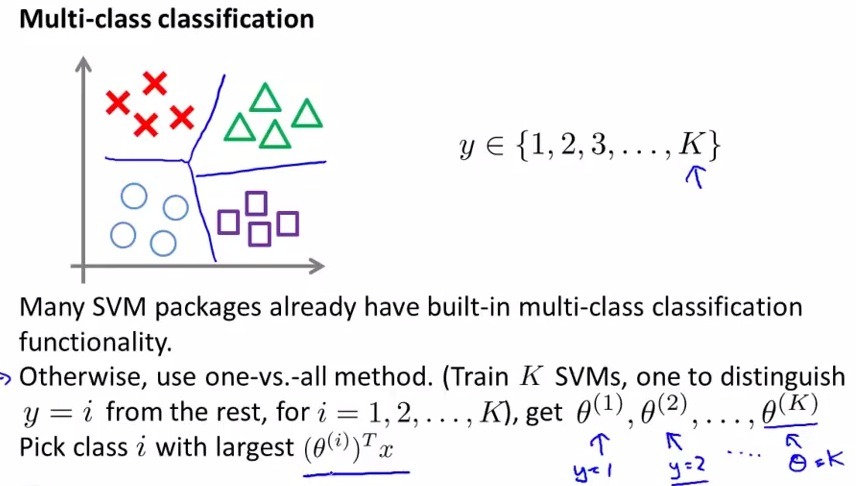• How to use SVM wisely in multiclass classification, is actually by using the built-in muticlass classification method that already inside whatever software package you use
• There's always high chance built-in SVM for multiclass classification
• Alternatively, use one vs all method. for each class, calculate theta1 to thetaK, with theta1 calculate for y=1, to thetaK calculate for y=k respectively. Then pick class i with largest hypothesis  among  theta.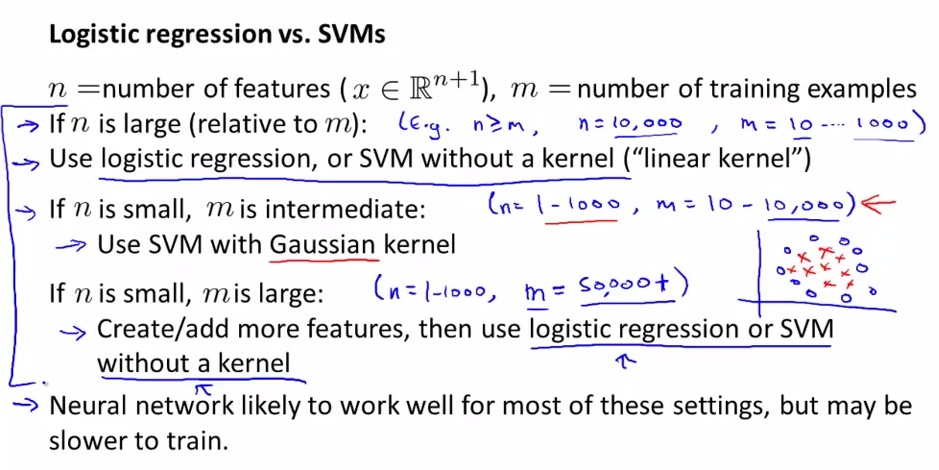• When do we use between these two algorithm?
• So many features without less data, then linear should be enough, because logistic will be hard, only increase complexity, and thus prone to overfitting, especially with only smaller training example.
• The second condition is where SVM with Gaussian kernel outshine all other algorithm.
• The third one is where SVM Gaussian kernel tends to fall in, even if we use software package. This is is the case where we talked earlier, that the number of parameters will match number of training example. So huge training example will increase complexity of calculation significantly.
• logistic regression or SVM without kernel perform similar and have result similarity. Only in special case where one perfom better than the other.
• Sometimes SVM built in package better than neural network, especially in specified regime mentioned above.
• SVM also perfom complex non-linear function as convex, as it always found global optima, so need to worry about it found local optima.

• SUMMARY
• At beginning feeling vague of which the algorithm to use
• Still widely recognized as one of the most popular powerful learning algorithm
• Logistic Regression and Neural Networks are widely used for learning algorithm
• Three algorithms (Logistic Regression, Neural Networks, SVM) alone in arsenal could be build state-of-the-art machine learning systems